# Angol nyelvű matematika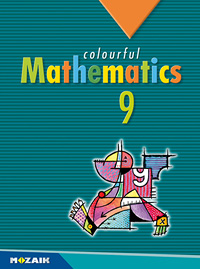MS-6309

## Colourful Mathematics 9.

9. évfolyam, 2. kiadás (2020. 08. 18.)
Mozaik Kiadó
méret: 170x240 mm
terjedelem: 276 oldal
tanterv: NAT 2012
4 980 Ft
3 984 Ft (20% osztálykedvezménnyel)*
Kosárba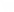* Legalább 15 példány megrendelése esetén 20% kedvezményt tudunk biztosítani 2021.03.31 és 2021.10.15 között.
 A kiadvány az MS-2309 Sokszínű matematika 9. c. kötet angol nyelvű változata. This book is the English version of the Hungarian market leader textbook titled Sokszínű matematika 9.
Kapcsolódó kiadványok
 A kiadvány digitális változata a könyvben levő kóddal ingyenesen elérhető*A kiadvány hátsó borítójának belső oldalán található egyedi kóddal a kiadvány digitálisan is elérhető. Az aktivált kódokkal DÍJMENTES hozzáférést biztosítunk a kiadvány mozaWeb Home változatához az aktiválástól számított minimum egy éves időtartamra. A kódok csak egyszer aktiválhatók.
Mintaoldalak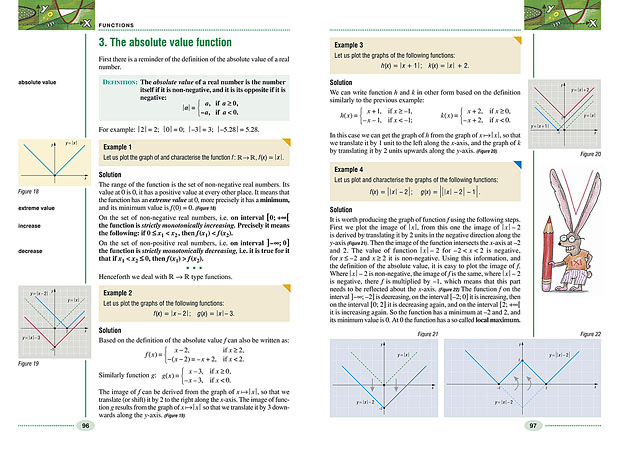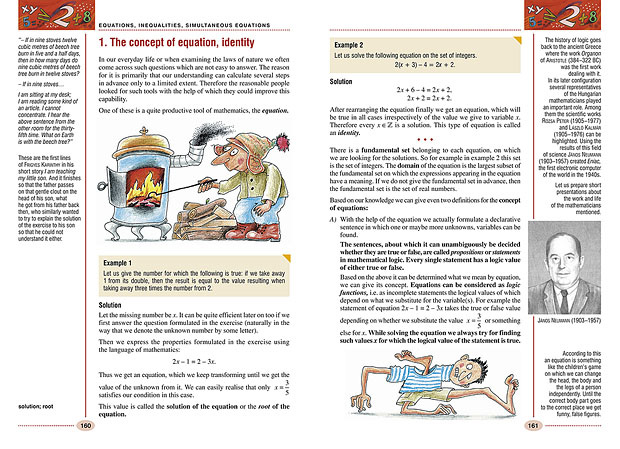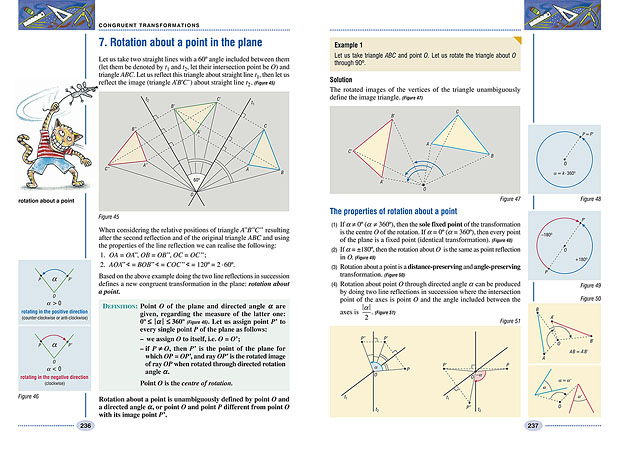Tartalomjegyzék
 Combinatorics, sets 10 1. The meaning of expressions in the mathematical language 10 2. Let us count up! 15 3. Sets 21 4. Set operations 26 5. Order of sets, inclusion-exclusion principle 32 6. Number lines, intervals 36 7. Graphs 38 Algebra and arithmetics 44 1. Usage of letters in mathematics 44 2. Exponentiation 48 3. Exponentiation to integer index 52 4. Standard index form of numbers 55 5. Integral expressions (polynomials) 58 6. Special algebraic products 60 7. Methods of factorisation 66 8. Operations with algebraic fractions 68 9. Divisibility 74 10. Greatest common divisor (GCD), least common multiple (LCM) 80 11. Number systems 83 Functions 88 1. The Cartesian coordinate system, point sets 88 2. Linear functions 92 3. The absolute value function 96 4. The quadratic function 102 5. The square root function 106 6. Linear fractional functions 110 7. The integral part, the fractional part and the algebraic sign function (higher level courseware) 116 8. More examples of functions (higher level courseware) 120 9. Systematization of function transformations 124 Triangles, quadrilaterals, polygons 128 1. Points, straight lines, planes and their mutual position . 128 2. A few basic geometric concepts (reminder) 129 3. About the triangles (reminder) 133 4. The relation between the sides and the angles of the triangle 135 5. The relation between the sides of a right-angled triangle 136 6. About the quadrilaterals (reminder) 139 7. About the polygons 143 8. Special point sets . 145 9. The inscribed circle of a triangle 149 10. The circumscribed circle of a triangle 151 11. Thales’ theorem and some of its applications . 153 12. Circumscribed quadrilaterals, circumscribed polygons (higher level courseware) 157 Equations, inequalities, simultaneous equations 160 1. The concept of equation, identity 160 2. Solving equations graphically 164 3. Solving equations with examining the domain and the range 166 4. Solving equations with factorisation 169 5. Solving equations with elimination, with the “balance method” 173 6. Inequalities 177 7. Equations and inequalities containing absolute value 182 8. Parametric equations (higher level courseware) 188 9. Solving problems with equations I 191 10. Solving problems with equations II 195 11. First-order simultaneous equations (system of equations) in two variables 199 12. Solving problems with simultaneous equations (systems of equations) 204 13. Linear systems of equations in more than two unknowns (higher level courseware) 209 14. Practical exercises 213 Congruent transformations 216 Congruent transformations 1. The concept of geometric transformation, examples of geometric transformations 216 2. Line reflection (reflection about a straight line) in the plane 218 3. Axially symmetric figures 221 4. Point reflection in the plane 225 5. Centrally symmetric figures 228 6. Applications of point reflection 231 7. Rotation about a point in the plane 236 8. Applications of rotation about a point I . 239 9. Applications of rotation about a point II 244 10. Parallel translation. Vectors 246 11. Operations with vectors 251 12. Congruence of figures 256 Statistics 260 1. The representation of data 260 2. The description of data 264
A kiadvány bevezetője
 Guide to use the course book. The notations and highlights used in the book help with acquiring the courseware. - The train of thought of the worked examples show samples how to understand the methods and processes and how to solve the subsequent exercises. - The most important definitions and theorems are denoted by colourful highlights. - The parts of the courseware in small print and the worked examples noted in claret colour help with deeper understanding of the courseware. These pieces of knowledge are necessary for the higher level of graduation. - Figures, the key points of the given lesson, review and explanatory parts along with interesting facts of the history of mathematics can be found on the margin. The difficulty level of the examples and the appointed exercises is denoted by three different colours: Yellow: drilling exercises with basic level difficulty; the solution and drilling of these exercises is essential for the progress. Blue: exercises the difficulty of which corresponds to the intermediate level of graduation. Claret: problems and exercises that help with preparing for the higher level of graduation. These colour codes correspond to the notations used in the Colourful mathematics workbooks of Mozaik Education. The workbook series contains more than 3000 exercises which are suitable for drilling, working on in lessons and which help with preparing for the graduation. The end results of the appointed exercises can be found on the following website: www.mozaik.info.hu. Website www.mozaweb.hu offers more help material for processing with the course book.

© MOZAIK KIADÓ - SZEGED, 2021 | Mobil